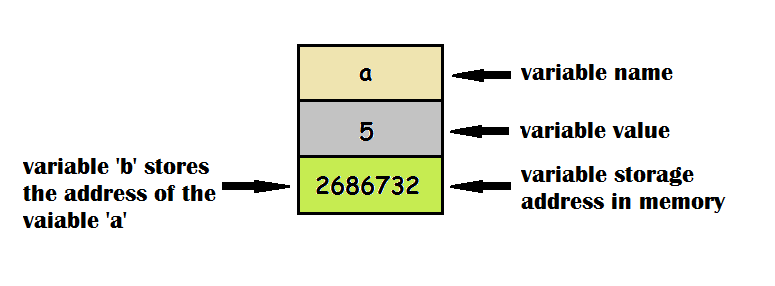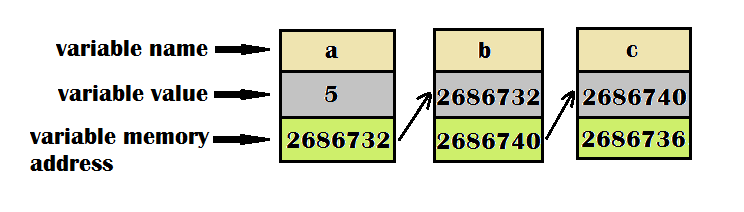# Pointers and its use in C Programming Language

Pointers are variables that contain the memory address of another variable. It enables the programmer to directly access the memory location and hence the value stored at it.

C programming allows a programmer to make use of pointer which is a very powerful tool for them. In the process of learning C Programming, one should be familiar with the use of pointers because there are some tasks that actually becomes easy with the use of pointers while some other tasks like dynamic memory allocation, which is just impossible without the use of pointers, so pointers actually play an important role in C programming. The following diagram illustrates the concept:In this figure, we see that a variable ‘a‘ stores the value ‘5‘ and another variable ‘b‘ stores the address value of variable ‘a‘ which is ‘2686732‘. Note that the address allocated to the variable ‘a‘ can be any random memory address and it might change while operating on a different system or when running different instances of the program or running it multiple times.

C language makes the use of certain operators to make it possible for the programmer to access the memory locations of a variable. In the following sections, we shall see the use of these operators.

### The ‘&’ (ampersand) operator

We have been using scanf() expression so frequently in all our previuos tutorials. Which contains the ‘ampersand‘ operator or ‘&’ which refers to the ‘address of’’ the variable. To directly access the memory location of the variable we can use this operator. The operator is written as a prefix to the variable whose address is to be found. Here is a program that shows how the above task can be done:

```#include <stdio.h>
int main() {
int a = 5; // assigning value to an integer variable
printf("The address of %d is: %u", a,
&a); //&a returns the address of the variable a
return 0;
}

```
```Output:-
The address of 5 is 2686732
```

### The ‘*’ (indirection) operator

Another important part of pointers is the ‘*’ operator or the ‘value at address’ operator. It returns the value stored at the particular address. It is also known as the ‘indirection’ operator. The following program shall show the use of the ‘*‘ operator in C Programming.

```#include <stdio.h>
int main() {
int a = 5;                                // a simple variable declaration
printf("The value of a is: %d\n", *(&a)); // it prints the same value as a
printf("The address of a is :%u",
&a); // printing address of the variable a in the memory
return 0;
}

```
```Output:-
The value of a is: 5
The address of a is :2686732
```

We see here that *(&a) yields the same result as ‘a‘ because the ‘*‘ operator returns the value at the address given by ‘&a‘.

### Declaration of Pointers

As we have already seen that pointers are variables so like other variables pointers too need to be declared. The ‘*’ operator is used while declaring a pointer. The syntax of the declaration is given below:

char *j;
int *i;
double *d;
float *f;

The address of any variable ought to be a number. Here we see that the variable *j has been declared as char. The reason behind this is that *j refers to the value at the address given by variable ‘j‘. So here the value at address ‘j‘ is a character value, hence, it is declared as a char.

### Pointer to a pointer variable

For every variable declared, a specific memory address is allocated to it for storage of data. In the case of pointers that data is the address of another variable. Now, we can consecutively access the memory address of the pointer by declaring another pointer variable to store the address of the pointer variable. The declaration is done as follows:

int a=5, *b, **c;

Here b = &a and c = &b.

This procedure stores the address of the variable ‘a‘ in ‘b‘ and the address of the variable ‘b‘ in ‘c‘. Look at the image below to understand the concept:### Program to show the use of pointers:

The program below shows the use of pointers and also the pointer to a pointer variable:

```#include <stdio.h>

int main() {
int a = 5, *b, **c; /* *b used in declaration  of a pointer. It gives the
value at the address stored in b. **c is used for the declaration of another
pointer. It points to the address of variable 'b' */

b = &a; // storing address of 'a' in variable 'b'
c = &b; // storing address of 'b' in variable 'c'

printf("The value of a is: %d\n",
*b); // it prints the value of 'a' stored in the location given by 'b'
printf("The address of a is :%u\n",
&a); // printing address of the variable 'a' in the memory
printf("The value of b is: %d\n", *c); // prints value stored at 'b'
printf("The address of b is: %u\n",
c); // prints the address of 'b' stored in variable 'c'
printf("The value of c is: %d\n",
&b); // prints the value of 'c' which is the address of variable 'b'
printf("The address of c is: %u\n", &c); // prints the address of variable 'c'

return 0;
}

```
```Output:-
The value of a is: 5
The address of a is :2686732
The value of b is: 2686732
The address of b is: 2686740
The value of c is: 2686740
The address of c is: 2686736
```

Note – The values of address shown in these examples will be different from what you will get while running your program.

An investment in knowledge always pays the best interest. Hope you like the tutorial. Do come back for more because learning paves way for a better understanding.

Do not forget to share and Subscribe.

Keep Coding!! Happy coding!! 🙂

### Recommended -

Subscribe
Notify of